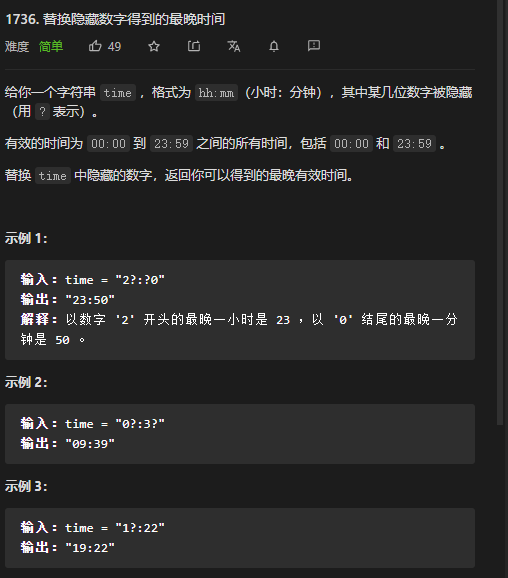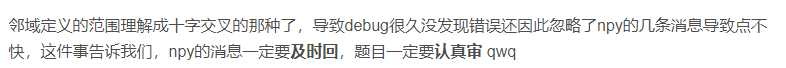# leetcode 1736WA了也不能不回消息吧(不过不是这个题)``````
#include<iostream>
#include<vector>
#include <queue>
#include <algorithm>
using namespace std;
//还是要注意一点就是string中的每一位都是char类型，搞数字的时候要  -‘0‘

class Solution {
public:
char if_time1_digit(string time){//return the number time should be
if(time-‘0‘>3)
return ‘1‘;
else{
return ‘2‘;
}
}
char if_time0_digit(string time){//return the number time should be
if(time==‘2‘)
return ‘3‘;
else{
return ‘9‘;
}
}
string maximumTime(string time) {//00:00
//之前遇见过这个题，无数if的叠加罢了。
//也是某种意义上的贪心
//其实后两个数跟前面没有什么关系，直接搞就行
//前两个数彼此有制约
/*
* 第一个数如果是二，第二个数只能是1 2 3
* 第二个数如果超过3，第一个数只能是1
* 如果两个数都没有，那就23（你可别傻到两个都取彼此的最大，29?）
* 那就，枚举问号在哪里吧！
*/
//前两个
if (time == ‘?‘ && time == ‘?‘) {
time = ‘2‘;
time = ‘3‘;
} else if (time == ‘?‘ && time != ‘?‘){//不要再犯以前的错误啦！你time和time可能已经更改过了，不要用if让它再进来了
time=if_time1_digit(time);

}else if(time == ‘?‘ && time != ‘?‘){
time=if_time0_digit(time);
}

if(time==‘?‘){
time=‘5‘;
}

if(time==‘?‘){
time=‘9‘;
}

return time;

}
};
``````

leetcode 1736

(0)
(0)